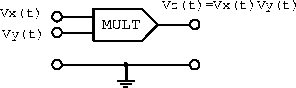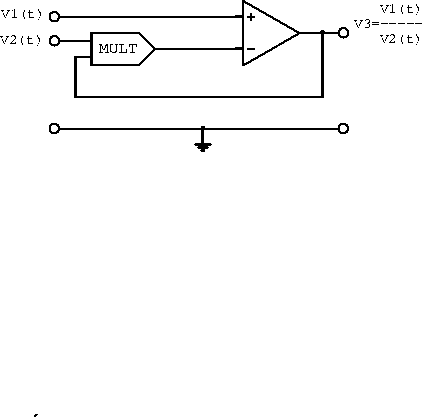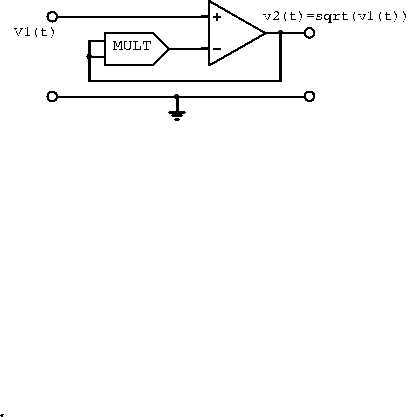Next: Differential Amplifiers Up: Ideal Amplifier Approximation Previous: Active Filters

## General Feedback Elements

The feedback elements in an operation amplifier design can be more complicated than a simple resistor and capacitor. An interesting feedback element is the analog multiplier as defined in figure 6.15.Figure 6.15:  Five-terminal network that performs the multiplication operation on two voltage signals.

The multiplier circuit itself can be thought of as another op-amp with a feedback resistor whose value is determined by a second input voltage. Multiplication circuits with the ability to handle input voltages of either sign (four-quadrant multipliers) are available as integrated circuits and have a number of direct uses as multipliers. But when used in a feedback loop around an operational amplifier, other useful functional forms result.

The circuit of figure 6.16 gives an output that is the ratio of two signals, whereas the circuit of figure 6.17 yields the analog square-root of the input voltages.Figure 6.16:  A multiplier as part of the feedback loop that results in the division operation.Figure 6.17:  A multiplier as part of the feedback loop that results in the square-root operation.

Doug Gingrich
Tue Jul 13 16:55:15 EDT 1999